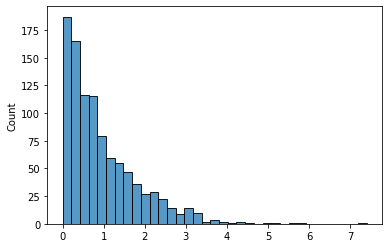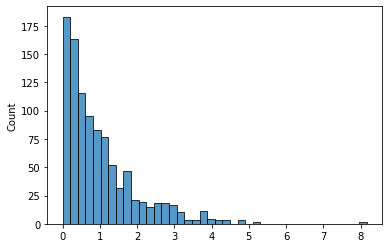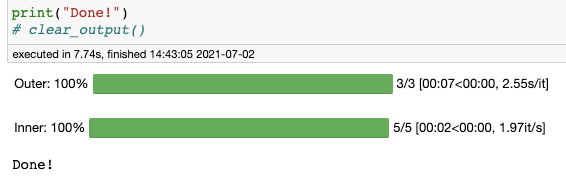# Jupyter notebooks

Jupyter is an open-source tool for executing Python code in an interactive notebook environment.

## Configuration

### Boilerplate

This is the boilerplate code I use to initialize every notebook.

You can add boilerplate imports to ~/.ipython/profile_default/startup/0_notebook_defaults.py to be executed every time the kernel is initialized.

import os, sys
import datetime as dt
import numpy as np
import pandas as pd
import matplotlib.pyplot as plt
import seaborn as sns

%matplotlib inline

from IPython.core.interactiveshell import InteractiveShell
InteractiveShell.ast_node_interactivity = 'all'  # display all output cells

from IPython.core.display import display, HTML
display(HTML("<style>.container { width:100% !important; }</style>"))  # make full width

pd.set_option('float_format', lambda x: '%.3f' % x)
pd.set_option('display.max_rows', 200)
np.set_printoptions(suppress=True, linewidth=180)


### SQL syntax highlighting

Add the following lines to ~/.jupyter/custom/custom.js:

IPython.notebook.events.one('kernel_ready.Kernel',
function(){
IPython.CodeCell.config_defaults
.highlight_modes['magic_text/x-mysql'] = {'reg':[/^%%sql/]} ;
IPython.notebook.get_cells().map(
function(cell){
if (cell.cell_type == 'code'){
cell.auto_highlight();
}
}) ;
}) ;


## Features

### Suppress output

Add ; to the end of the line, useful when you want to prevent text output when plotting.

data = np.random.exponential(size=1000)
sns.histplot(data, kde=False)

<AxesSubplot:ylabel='Count'>data = np.random.exponential(size=1000)
sns.histplot(data, kde=False);### Check python version

from platform import python_version

python_version()

'3.8.10'


## Tips & tricks

### Idempotent pip installs

If your notebook has dependecies, you can make it “one-click runnable” using !pip install -Uqq module.

This will silently install or upgrade a pip package, showing no output unless an error occurs.

### Progress bars w/ tqdm

from time import sleep
from tqdm.notebook import tqdm
from IPython.display import clear_output

iters_outer = 3
iters_inner = 5
for i in tqdm(range(iters_outer), desc='Outer'):
for j in tqdm(range(iters_inner), desc='Inner', leave=(i==iters_outer-1)):
sleep(0.5)

print("Done!")
clear_output()


The tqdm progress bars do not render properly when this notebook is converted to markdown, but below is a screenshot of what it looks like in-notebook.## Magics

Our test function

• Our function sleeps for $X \sim \text{Unif}(0, 1)$ seconds.
• So we expect an average latency of $E[ X ] = 0.5$ seconds, plus perhaps a tiny bit of overhead on calling the function.
• Our expected standard deviation is $S_X = \sqrt{\text{Var}(x)} = \sqrt{\tfrac{1}{12}(b-a)^2} \approx 0.28$.
from time import sleep
from random import random

def my_func():
sleep(random())  # Random number in range [0, 1]
return True


### Timing execution

#### timeit magic

• Useful one-liner for calculating average execution time.
• Does not print return value of function.

Arguments

• Will execute the function a total of n*r times
• The -n argument dictates how many loops from which to take the lowest time.
• The -r dictates how many runs, which are used for the ± stats.
%timeit my_func()

565 ms ± 69.1 ms per loop (mean ± std. dev. of 7 runs, 10 loops each)

%timeit -r 100 -n 1 my_func()

The slowest run took 829.94 times longer than the fastest. This could mean that an intermediate result is being cached.
552 ms ± 302 ms per loop (mean ± std. dev. of 100 runs, 1 loop each)

%timeit -r 1 -n 100 my_func()

507 ms ± 0 ns per loop (mean ± std. dev. of 1 run, 100 loops each)

%timeit -r 10 -n 10 my_func()

576 ms ± 79.9 ms per loop (mean ± std. dev. of 10 runs, 10 loops each)

%timeit -r 50 -n 2 my_func()

The slowest run took 7.53 times longer than the fastest. This could mean that an intermediate result is being cached.
477 ms ± 168 ms per loop (mean ± std. dev. of 50 runs, 2 loops each)


So the best approach is to call timeit with arguments -r <n> -n 1, since otherwise it will underestimate the variability in run times.

#### Line profiling

!pip install -Uqq line_profiler

%lprun -f my_func my_func()


### Memory profiling

Can be used for:

• Functions
• Objects → sys.getsizeof(x) is not accurate, because it works for built-ins but not for custom-defined objects.
!pip install -Uqq memory_profiler


peak memory: 51.43 MiB, increment: 0.00 MiB

%debug magic (docs)